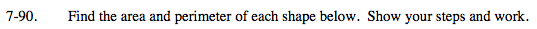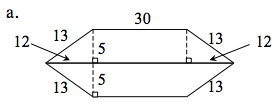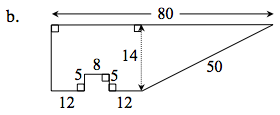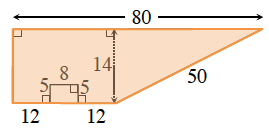### Home > MC2 > Chapter Ch7 > Lesson 7.2.3 > Problem7-90

7-90.Perimeter: Add the lengths of each of he sides around the outside of the figure.

13 + 30 + 13 + 13 + 30 + 13 = 112 units

The shape consists of two trapezoids of equal size. Find the area of one trapezoid and double it to find the area of the entire shape.

The area of a trapezoid can be found with the following formula:

$A=\frac{1}{2}(\text{base}_1+\text{base}_2)(\text{height})$

420 square unitsYou can use the equation for the area of a trapezoid to find the total area.

The trapezoid is highlighted.
Notice that there is a rectangular section missing from the trapezoid.
We will need to find that area as well.744 sq. units.